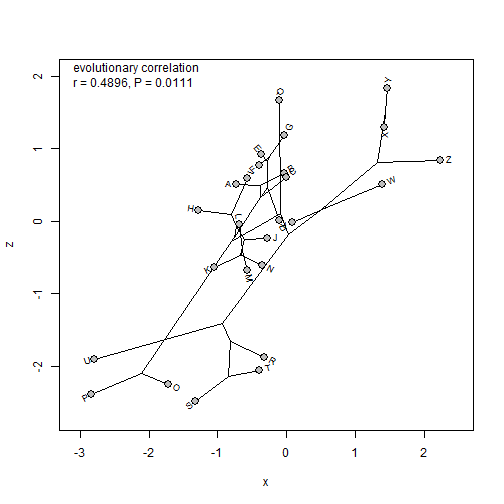## Wednesday, August 30, 2017

### Pearson correlation with phylogenetic data

Today I was asked the following question:

“I am interested in testing the correlation between 2 traits taking into account for the phylogenetic structure in my dataset. PGLS is a good way to test it, but I was wondering if a simple correlation test (using a Pearson r) would be possible. Your `phyl.vcv` computes a phylogenetic trait variance-covariance matrix between two variables. Can I use this matrix to compute a phylogenetic Pearson r value? If so, can this r-value be used to compute the statistic to test the significance of the correlation (with n-2 df in a t distribution)?”

The question was also posted here.

The answer is basically, yes & yes - but it is not strictly necessary as PGLS will give us the same p-value. Let's see how:

``````library(phytools)
## our data
tree
``````
``````##
## Phylogenetic tree with 26 tips and 25 internal nodes.
##
## Tip labels:
##  A, B, C, D, E, F, ...
##
## Rooted; includes branch lengths.
``````
``````X
``````
``````##              x           y           z
## A -0.723942909  0.96553388  0.50467165
## B -0.034600412  1.48143249  0.66533528
## C -0.001282309  0.62094719  0.60352347
## D -0.100971061  1.23102030  0.01866007
## E -0.363619319  0.06831715  0.91782821
## F -0.400834652 -0.07312087  0.77584466
## G -0.037785409  0.18406320  1.18509956
## H -1.284112703  0.26824899  0.15203366
## I -0.573447373  1.56312743  0.58464867
## J -0.275070942  0.05165640 -0.24120290
## K -1.050699705  0.31715393 -0.63071969
## L -0.689968116  0.22713074 -0.04087866
## M -0.572979102  0.16002629 -0.67389194
## N -0.353661739  0.31157683 -0.60997825
## O -1.720295244  0.57079006 -2.24647340
## P -2.830254149  0.78574612 -2.39094415
## Q -0.104129552  0.93488329  1.66983162
## R -0.324883255  1.00993141 -1.87523279
## S -1.330114250  0.53192888 -2.47716184
## T -0.394635253  0.84544343 -2.05151552
## U -2.788040191  0.75579827 -1.90934711
## V  0.076717481 -1.13500947 -0.01313445
## W  1.388265725 -1.15038331  0.50393587
## X  1.423667629 -1.41685533  1.29662644
## Y  1.459867791 -0.53755989  1.83210421
## Z  2.235835060 -1.28003540  0.84061852
``````

First, let's compute the evolutionary (phylogenetic) correlation between `x` & `y`:

``````## our covariance matrix
obj<-phyl.vcv(X,vcv(tree),1)
obj\$R
``````
``````##            x          y         z
## x  0.6810667 -0.0592844 0.3212055
## y -0.0592844  0.4238996 0.0413417
## z  0.3212055  0.0413417 0.6319725
``````
``````## correlation between x & y
r.xy<-cov2cor(obj\$R)["x","y"]
## t-statistic & P-value
t.xy<-r.xy*sqrt((Ntip(tree)-2)/(1-r.xy^2))
P.xy<-2*pt(abs(t.xy),df=Ntip(tree)-2,lower.tail=F)
P.xy
``````
``````##  0.5915613
``````

As noted before, this is the same P-value as we would obtain fitting a linear model using PGLS of `y~x` or `x~y`.

``````fit.yx<-gls(y~x,data=as.data.frame(X),correlation=corBrownian(1,tree))
anova(fit.yx)
``````
``````## Denom. DF: 24
##             numDF   F-value p-value
## (Intercept)     1 0.2645170  0.6117
## x               1 0.2957732  0.5916
``````
``````fit.xy<-gls(x~y,data=as.data.frame(X),correlation=corBrownian(1,tree))
anova(fit.xy)
``````
``````## Denom. DF: 24
##             numDF   F-value p-value
## (Intercept)     1 0.1812473  0.6741
## y               1 0.2957732  0.5916
``````

Now let's see an example in which a weak correlation exists between the two characters:

``````## correlation between x & z
r.xz<-cov2cor(obj\$R)["x","z"]
## t-statistic & P-value
t.xz<-r.xz*sqrt((Ntip(tree)-2)/(1-r.xz^2))
P.xz<-2*pt(abs(t.xz),df=Ntip(tree)-2,lower.tail=F)
P.xz
``````
``````##  0.01112811
``````
``````fit.zx<-gls(z~x,data=as.data.frame(X),correlation=corBrownian(1,tree))
anova(fit.zx)
``````
``````## Denom. DF: 24
##             numDF  F-value p-value
## (Intercept)     1 0.003181  0.9555
## x               1 7.566719  0.0111
``````
``````## visualize it
phylomorphospace(tree,X[,c("x","z")],node.size=c(0,0))
points(X[,c("x","z")],pch=21,cex=1.5,bg="grey")
text(-3.2,2,paste("evolutionary correlation\nr = ",round(r.xz,4),
", P = ",round(P.xz,4),sep="" ),pos=4)
``````Neat.

The data for this example were simulated as follows:

``````tree<-pbtree(n=26,tip.label=LETTERS)
X<-sim.corrs(tree,
vcv=matrix(c(1.0,0.0,0.3,
0.0,1.0,0.0,
0.3,0.0,1.0),3,3))
colnames(X)<-c("x","y","z")
``````

#### 1 comment:

1.Hey! Thanks for the super useful code!
I've found that it works well, but values change a lot depending on lambda values. How do you go about selecting lambda values for two traits? I tried a pgls, but it changes depending on which one is the response variable.

Cheers
Rob

Note: due to the very large amount of spam, all comments are now automatically submitted for moderation.